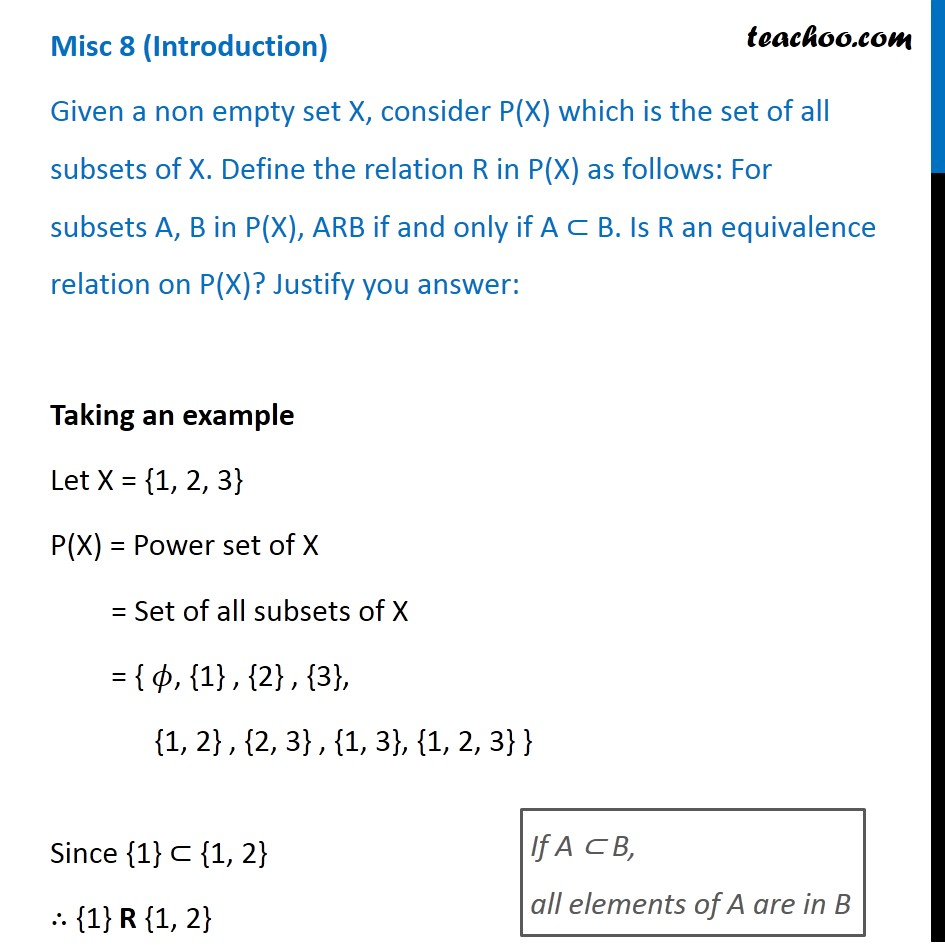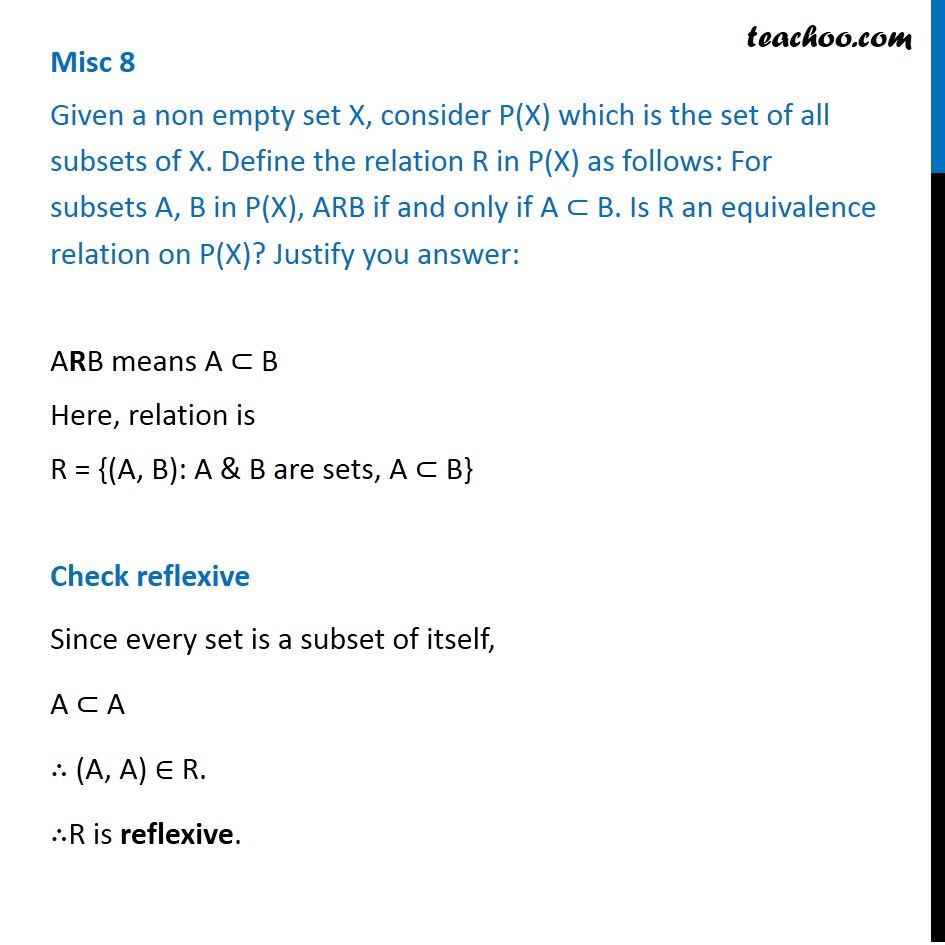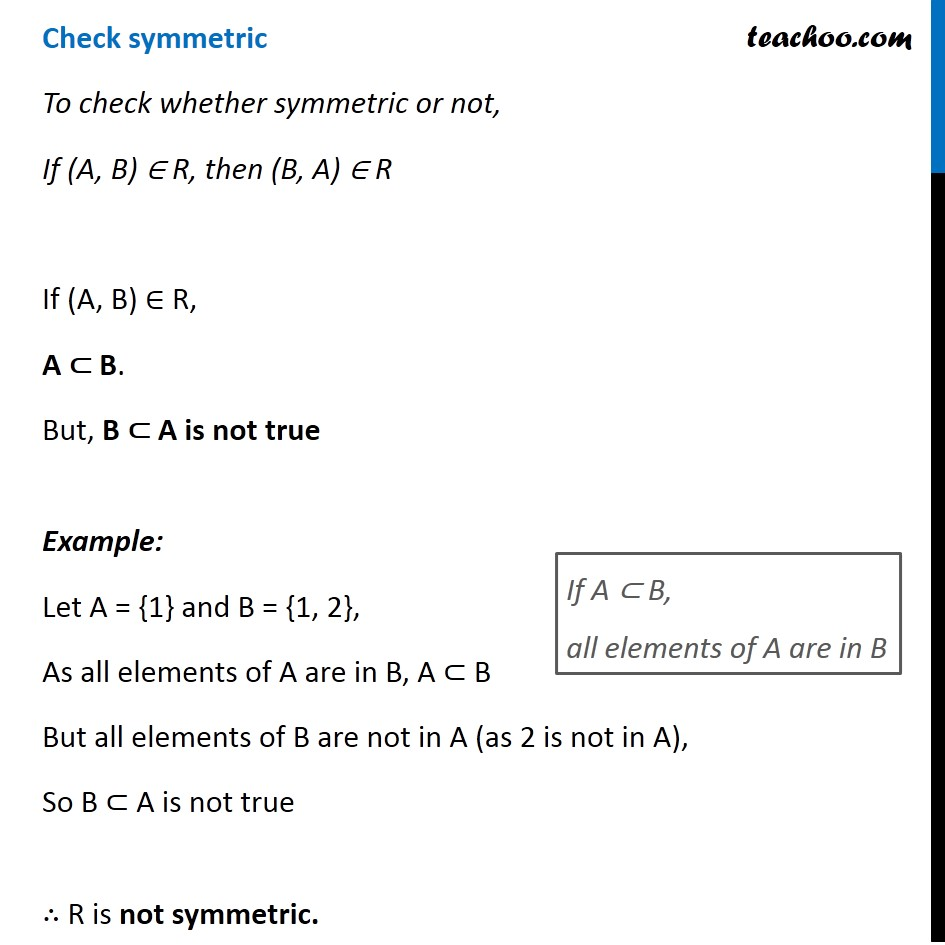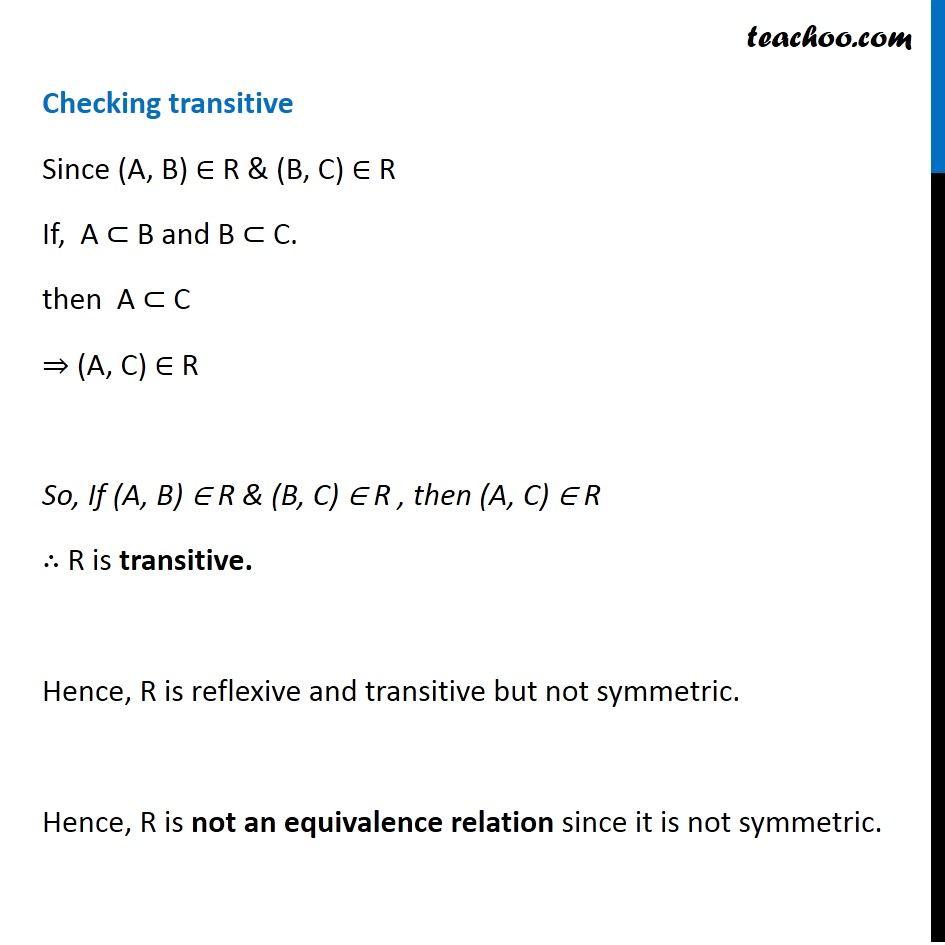To prove relation reflexive, transitive, symmetric and equivalent

Chapter 1 Class 12 Relation and Functions
Concept wiseIntroducing your new favourite teacher - Teachoo Black, at only ₹83 per month

### Transcript

Misc 8 (Introduction) Given a non empty set X, consider P(X) which is the set of all subsets of X. Define the relation R in P(X) as follows: For subsets A, B in P(X), ARB if and only if A ⊂ B. Is R an equivalence relation on P(X)? Justify you answer: Taking an example Let X = {1, 2, 3} P(X) = Power set of X = Set of all subsets of X = { 𝜙, {1} , {2} , {3}, {1, 2} , {2, 3} , {1, 3}, {1, 2, 3} } Since {1} ⊂ {1, 2} ∴ {1} R {1, 2} If A ⊂ B, all elements of A are in B Misc 8 Given a non empty set X, consider P(X) which is the set of all subsets of X. Define the relation R in P(X) as follows: For subsets A, B in P(X), ARB if and only if A ⊂ B. Is R an equivalence relation on P(X)? Justify you answer: ARB means A ⊂ B Here, relation is R = {(A, B): A & B are sets, A ⊂ B} Check reflexive Since every set is a subset of itself, A ⊂ A ∴ (A, A) ∈ R. ∴R is reflexive. Check symmetric To check whether symmetric or not, If (A, B) ∈ R, then (B, A) ∈ R If (A, B) ∈ R, A ⊂ B. But, B ⊂ A is not true Example: Let A = {1} and B = {1, 2}, As all elements of A are in B, A ⊂ B But all elements of B are not in A (as 2 is not in A), So B ⊂ A is not true ∴ R is not symmetric. If A ⊂ B, all elements of A are in B Checking transitive Since (A, B) ∈ R & (B, C) ∈ R If, A ⊂ B and B ⊂ C. then A ⊂ C ⇒ (A, C) ∈ R So, If (A, B) ∈ R & (B, C) ∈ R , then (A, C) ∈ R ∴ R is transitive. Hence, R is reflexive and transitive but not symmetric. Hence, R is not an equivalence relation since it is not symmetric.